Anúncio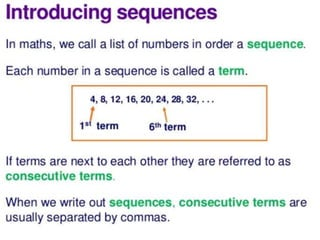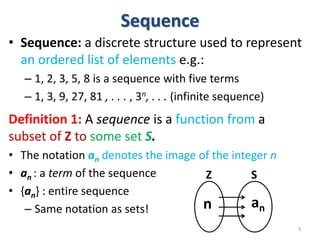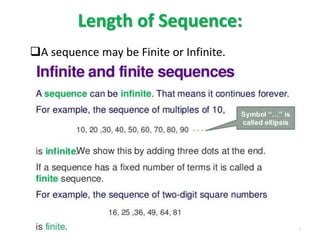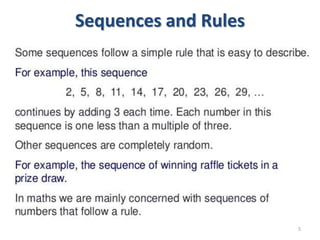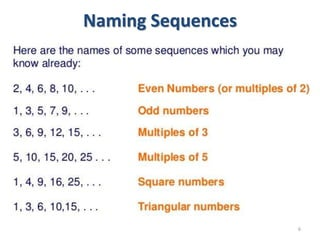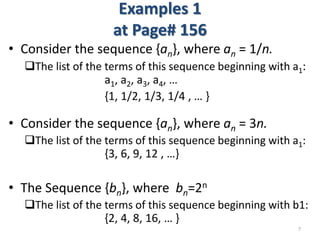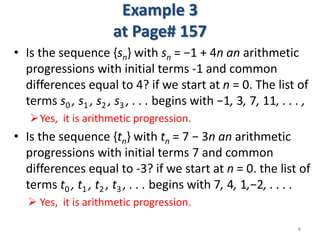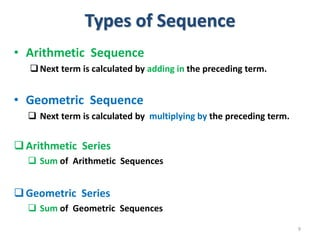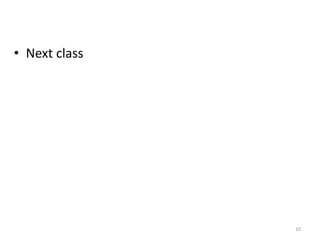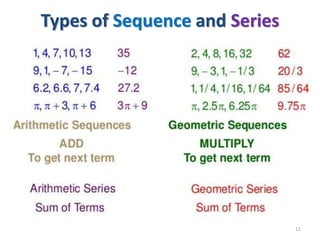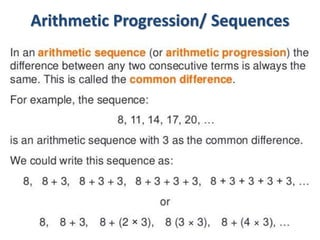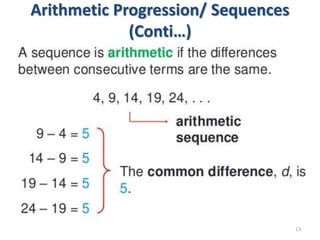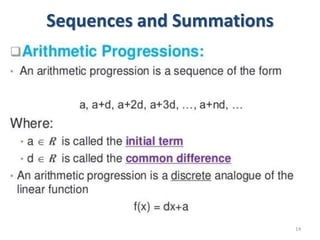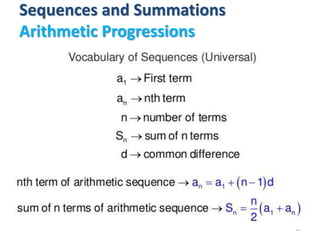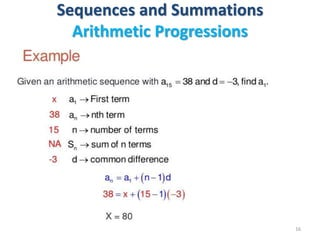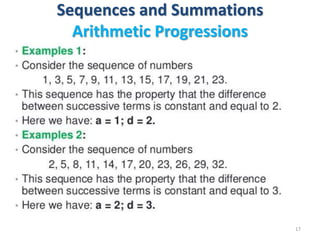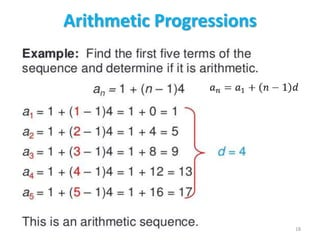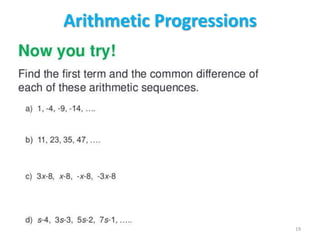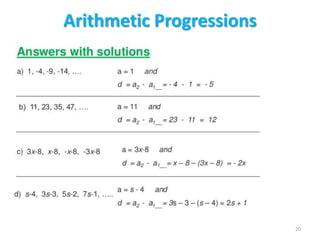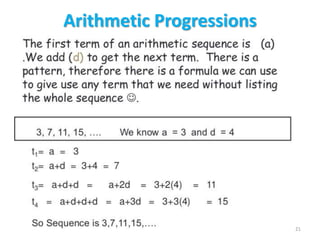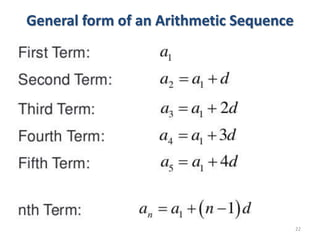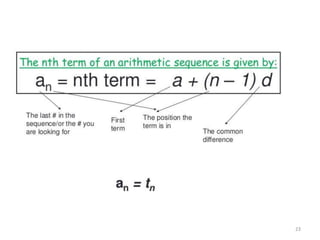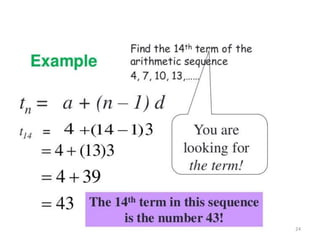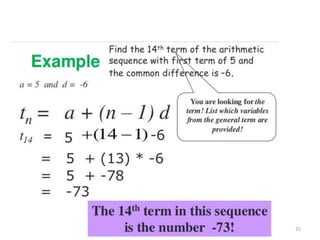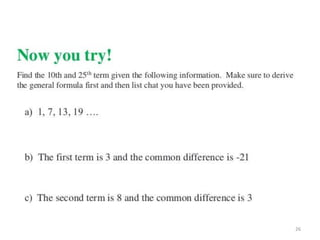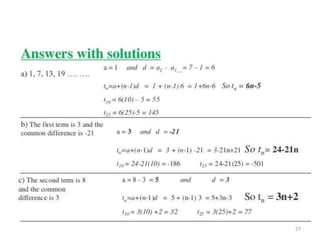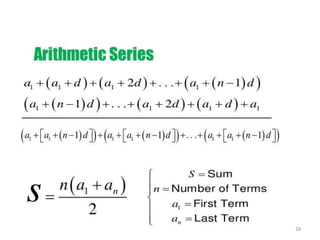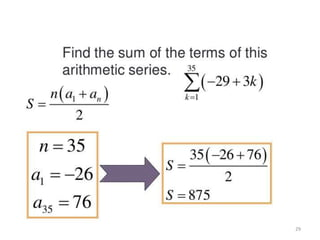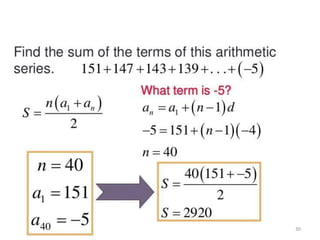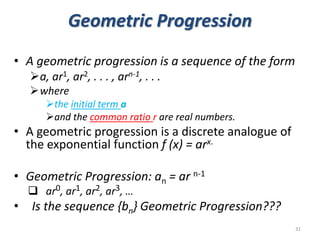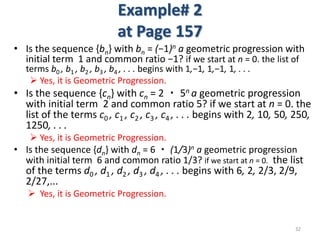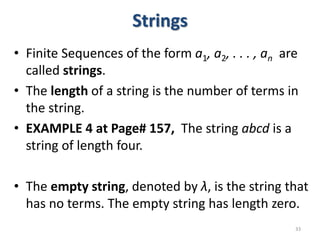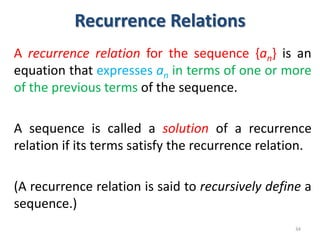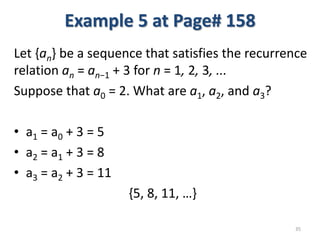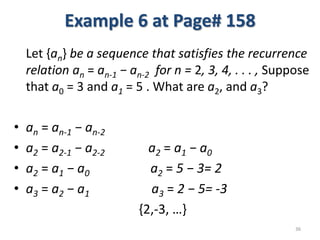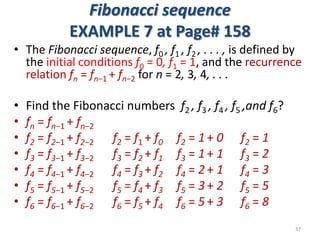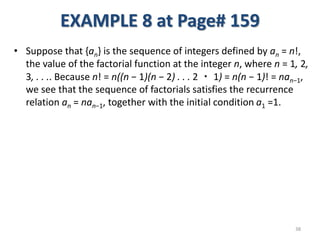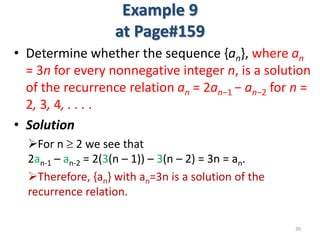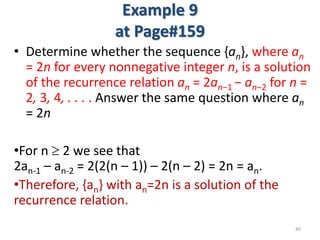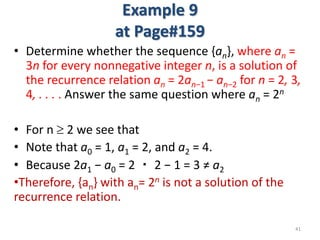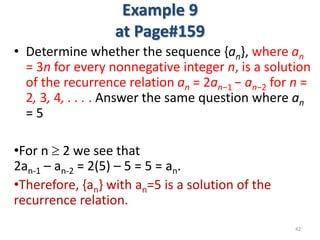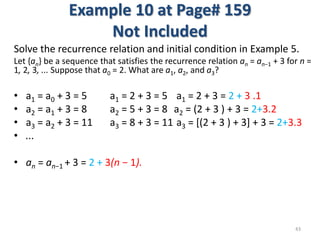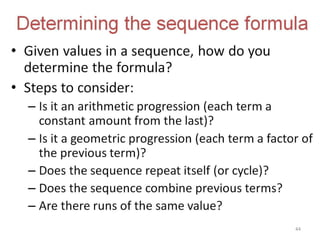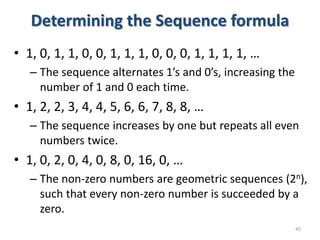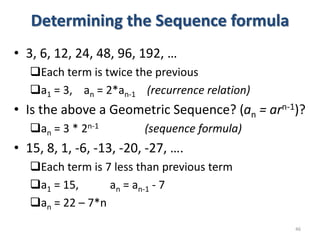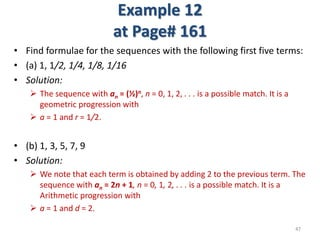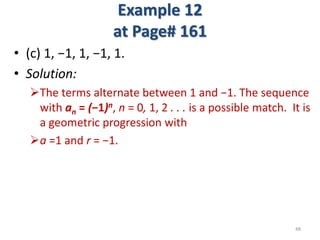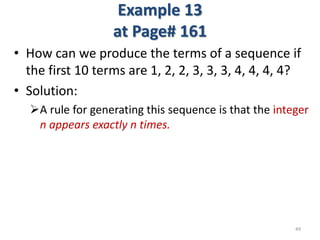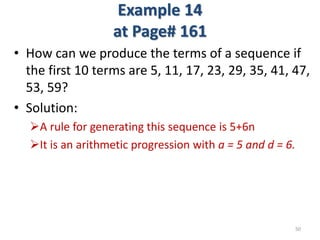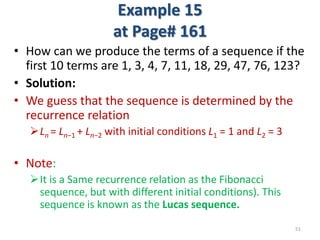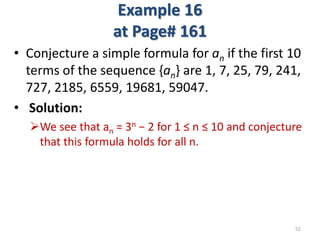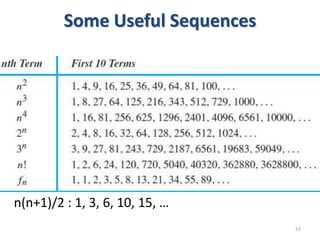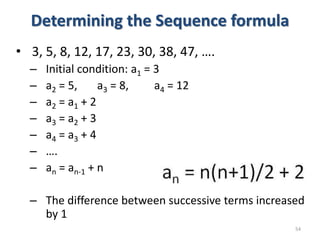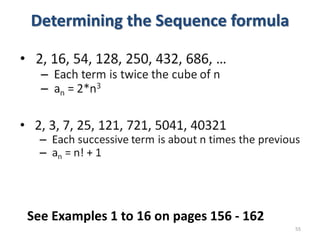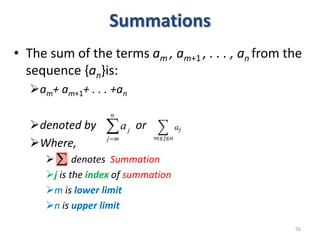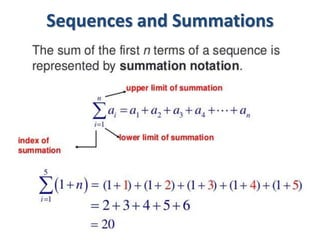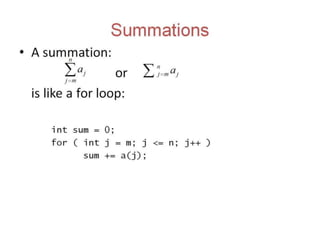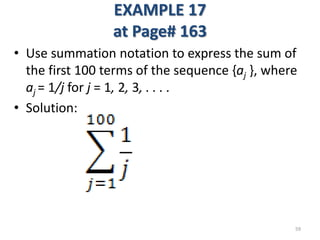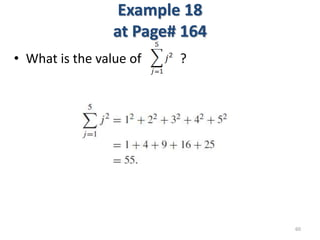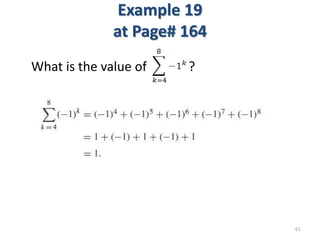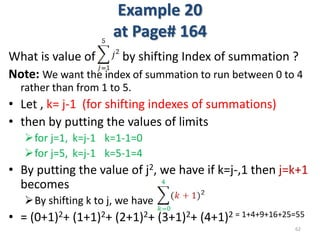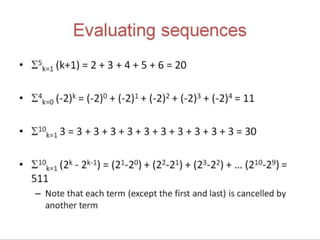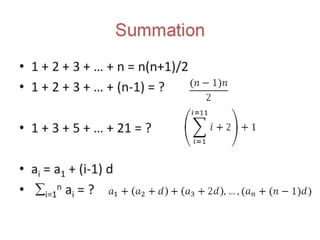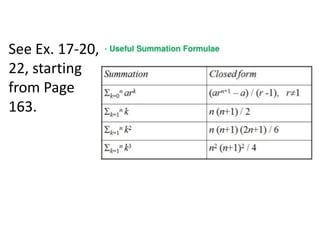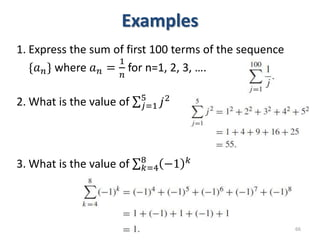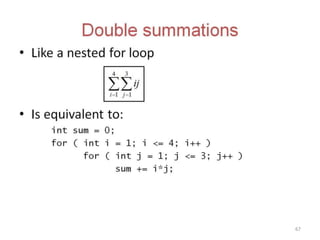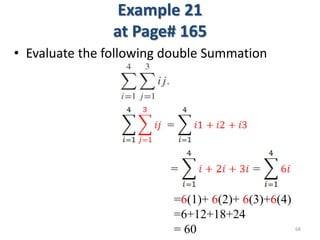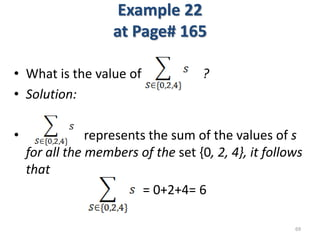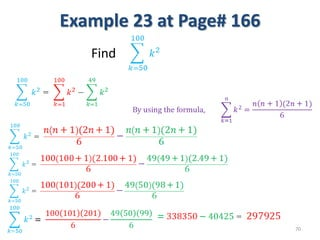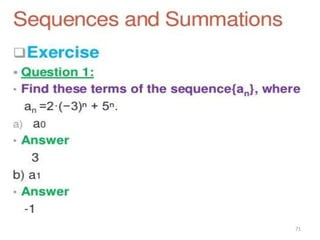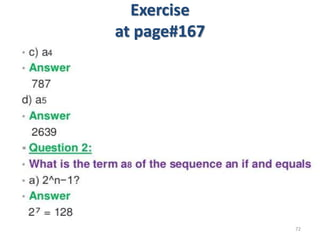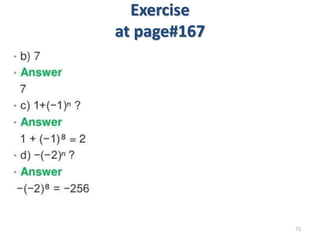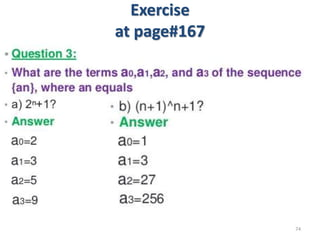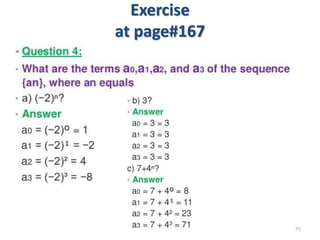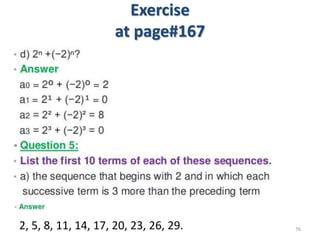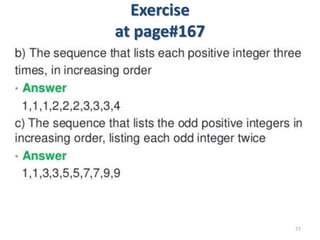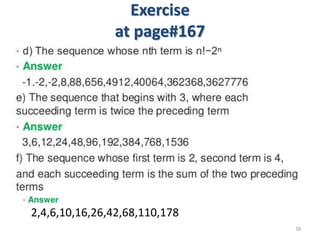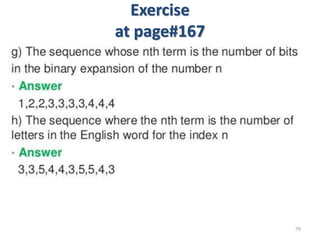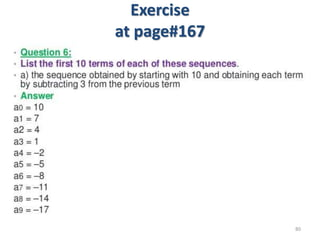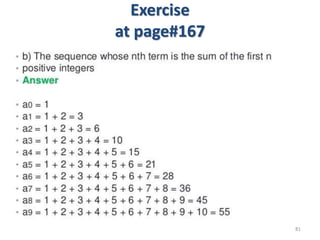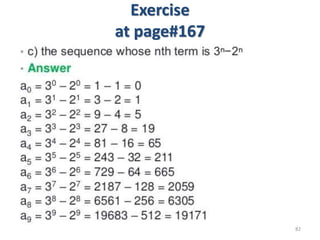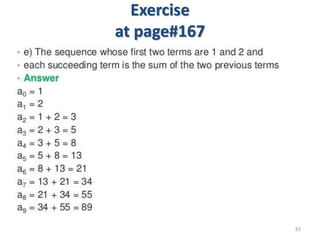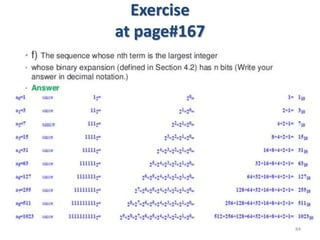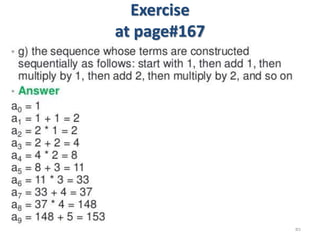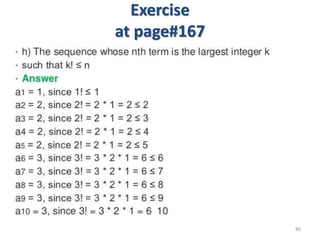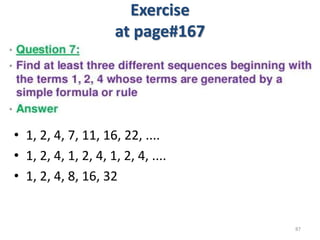1 de 87
Anúncio

### 10-Sequences and summation.pptx

1. Discrete Structures Sequences and Summations Dr. Muhammad Humayoun Assistant Professor COMSATS Institute of Computer Science, Lahore. mhumayoun@ciitlahore.edu.pk https://sites.google.com/a/ciitlahore.edu.pk/dstruct/ A lot of material is taken from the slides of Dr. Atif and Dr. Mudassir 1
2. 2
3. Sequence • Sequence: a discrete structure used to represent an ordered list of elements e.g.: – 1, 2, 3, 5, 8 is a sequence with five terms – 1, 3, 9, 27, 81 , . . . , 3n, . . . (infinite sequence) Definition 1: A sequence is a function from a subset of Z to some set S. • The notation an denotes the image of the integer n • an : a term of the sequence • {an} : entire sequence – Same notation as sets! 3 Z S n an
4. Length of Sequence: A sequence may be Finite or Infinite. 4
5. Sequences and Rules 5
6. Naming Sequences 6
7. Examples 1 at Page# 156 • Consider the sequence {an}, where an = 1/n. The list of the terms of this sequence beginning with a1: a1, a2, a3, a4, … {1, 1/2, 1/3, 1/4 , … } • Consider the sequence {an}, where an = 3n. The list of the terms of this sequence beginning with a1: {3, 6, 9, 12 , …} • The Sequence {bn}, where bn=2n The list of the terms of this sequence beginning with b1: {2, 4, 8, 16, … } 7
8. Example 3 at Page# 157 • Is the sequence {sn} with sn = −1 + 4n an arithmetic progressions with initial terms -1 and common differences equal to 4? if we start at n = 0. The list of terms s0 , s1 , s2 , s3 , . . . begins with −1, 3, 7, 11, . . . , Yes, it is arithmetic progression. • Is the sequence {tn} with tn = 7 − 3n an arithmetic progressions with initial terms 7 and common differences equal to -3? if we start at n = 0. the list of terms t0 , t1 , t2 , t3 , . . . begins with 7, 4, 1,−2, . . . .  Yes, it is arithmetic progression. 8
9. Types of Sequence • Arithmetic Sequence Next term is calculated by adding in the preceding term. • Geometric Sequence  Next term is calculated by multiplying by the preceding term. Arithmetic Series  Sum of Arithmetic Sequences Geometric Series  Sum of Geometric Sequences 9
10. • Next class 10
11. Types of Sequence and Series 11
12. Arithmetic Progression/ Sequences 12
13. Arithmetic Progression/ Sequences (Conti…) 13
14. Sequences and Summations 14
15. Sequences and Summations Arithmetic Progressions 15
16. Sequences and Summations Arithmetic Progressions 16
17. Sequences and Summations Arithmetic Progressions 17
18. Arithmetic Progressions 18
19. Arithmetic Progressions 19
20. Arithmetic Progressions 20
21. Arithmetic Progressions 21
22. General form of an Arithmetic Sequence 22
23. 23
24. 24
25. 25
26. 26
27. 27
28. 28
29. 29
30. 30
31. Geometric Progression • A geometric progression is a sequence of the form a, ar1, ar2, . . . , arn-1, . . . where the initial term a and the common ratio r are real numbers. • A geometric progression is a discrete analogue of the exponential function f (x) = arx. • Geometric Progression: an = ar n-1  ar0, ar1, ar2, ar3, … • Is the sequence {bn} Geometric Progression??? 31
32. Example# 2 at Page 157 • Is the sequence {bn} with bn = (−1)n a geometric progression with initial term 1 and common ratio −1? if we start at n = 0. the list of terms b0 , b1 , b2 , b3 , b4 , . . . begins with 1,−1, 1,−1, 1, . . .  Yes, it is Geometric Progression. • Is the sequence {cn} with cn = 2 ・ 5n a geometric progression with initial term 2 and common ratio 5? if we start at n = 0. the list of the terms c0 , c1 , c2 , c3 , c4 , . . . begins with 2, 10, 50, 250, 1250, . . .  Yes, it is Geometric Progression. • Is the sequence {dn} with dn = 6 ・ (1/3)n a geometric progression with initial term 6 and common ratio 1/3? if we start at n = 0. the list of the terms d0 , d1 , d2 , d3 , d4 , . . . begins with 6, 2, 2/3, 2/9, 2/27,...  Yes, it is Geometric Progression. 32
33. Strings • Finite Sequences of the form a1, a2, . . . , an are called strings. • The length of a string is the number of terms in the string. • EXAMPLE 4 at Page# 157, The string abcd is a string of length four. • The empty string, denoted by λ, is the string that has no terms. The empty string has length zero. 33
34. Recurrence Relations A recurrence relation for the sequence {an} is an equation that expresses an in terms of one or more of the previous terms of the sequence. A sequence is called a solution of a recurrence relation if its terms satisfy the recurrence relation. (A recurrence relation is said to recursively define a sequence.) 34
35. Example 5 at Page# 158 Let {an} be a sequence that satisfies the recurrence relation an = an−1 + 3 for n = 1, 2, 3, ... Suppose that a0 = 2. What are a1, a2, and a3? • a1 = a0 + 3 = 5 • a2 = a1 + 3 = 8 • a3 = a2 + 3 = 11 {5, 8, 11, …} 35
36. Example 6 at Page# 158 Let {an} be a sequence that satisfies the recurrence relation an = an-1 − an-2 for n = 2, 3, 4, . . . , Suppose that a0 = 3 and a1 = 5 . What are a2, and a3? • an = an-1 − an-2 • a2 = a2-1 − a2-2 a2 = a1 − a0 • a2 = a1 − a0 a2 = 5 − 3= 2 • a3 = a2 − a1 a3 = 2 − 5= -3 {2,-3, …} 36
37. Fibonacci sequence EXAMPLE 7 at Page# 158 • The Fibonacci sequence, f0 , f1 , f2 , . . . , is defined by the initial conditions f0 = 0, f1 = 1, and the recurrence relation fn = fn−1 + fn−2 for n = 2, 3, 4, . . . • Find the Fibonacci numbers f2 , f3 , f4 , f5 ,and f6? • fn = fn−1 + fn−2 • f2 = f2−1 + f2−2 f2 = f1 + f0 f2 = 1 + 0 f2 = 1 • f3 = f3−1 + f3−2 f3 = f2 + f1 f3 = 1 + 1 f3 = 2 • f4 = f4−1 + f4−2 f4 = f3 + f2 f4 = 2 + 1 f4 = 3 • f5 = f5−1 + f5−2 f5 = f4 + f3 f5 = 3 + 2 f5 = 5 • f6 = f6−1 + f6−2 f6 = f5 + f4 f6 = 5 + 3 f6 = 8 37
38. EXAMPLE 8 at Page# 159 • Suppose that {an} is the sequence of integers defined by an = n!, the value of the factorial function at the integer n, where n = 1, 2, 3, . . .. Because n! = n((n − 1)(n − 2) . . . 2 ・ 1) = n(n − 1)! = nan−1, we see that the sequence of factorials satisfies the recurrence relation an = nan−1, together with the initial condition a1 =1. 38
39. Example 9 at Page#159 • Determine whether the sequence {an}, where an = 3n for every nonnegative integer n, is a solution of the recurrence relation an = 2an−1 − an−2 for n = 2, 3, 4, . . . . • Solution For n  2 we see that 2an-1 – an-2 = 2(3(n – 1)) – 3(n – 2) = 3n = an. Therefore, {an} with an=3n is a solution of the recurrence relation. 39
40. Example 9 at Page#159 • Determine whether the sequence {an}, where an = 2n for every nonnegative integer n, is a solution of the recurrence relation an = 2an−1 − an−2 for n = 2, 3, 4, . . . . Answer the same question where an = 2n •For n  2 we see that 2an-1 – an-2 = 2(2(n – 1)) – 2(n – 2) = 2n = an. •Therefore, {an} with an=2n is a solution of the recurrence relation. 40
41. Example 9 at Page#159 • Determine whether the sequence {an}, where an = 3n for every nonnegative integer n, is a solution of the recurrence relation an = 2an−1 − an−2 for n = 2, 3, 4, . . . . Answer the same question where an = 2n • For n  2 we see that • Note that a0 = 1, a1 = 2, and a2 = 4. • Because 2a1 − a0 = 2 ・ 2 − 1 = 3 ≠ a2 •Therefore, {an} with an= 2n is not a solution of the recurrence relation. 41
42. Example 9 at Page#159 • Determine whether the sequence {an}, where an = 3n for every nonnegative integer n, is a solution of the recurrence relation an = 2an−1 − an−2 for n = 2, 3, 4, . . . . Answer the same question where an = 5 •For n  2 we see that 2an-1 – an-2 = 2(5) – 5 = 5 = an. •Therefore, {an} with an=5 is a solution of the recurrence relation. 42
43. Example 10 at Page# 159 Not Included Solve the recurrence relation and initial condition in Example 5. Let {an} be a sequence that satisfies the recurrence relation an = an−1 + 3 for n = 1, 2, 3, ... Suppose that a0 = 2. What are a1, a2, and a3? • a1 = a0 + 3 = 5 a1 = 2 + 3 = 5 a1 = 2 + 3 = 2 + 3 .1 • a2 = a1 + 3 = 8 a2 = 5 + 3 = 8 a2 = (2 + 3 ) + 3 = 2+3.2 • a3 = a2 + 3 = 11 a3 = 8 + 3 = 11 a3 = [(2 + 3 ) + 3] + 3 = 2+3.3 • ... • an = an−1 + 3 = 2 + 3(n − 1). 43
44. 44
45. Determining the Sequence formula • 1, 0, 1, 1, 0, 0, 1, 1, 1, 0, 0, 0, 1, 1, 1, 1, … – The sequence alternates 1’s and 0’s, increasing the number of 1 and 0 each time. • 1, 2, 2, 3, 4, 4, 5, 6, 6, 7, 8, 8, … – The sequence increases by one but repeats all even numbers twice. • 1, 0, 2, 0, 4, 0, 8, 0, 16, 0, … – The non-zero numbers are geometric sequences (2n), such that every non-zero number is succeeded by a zero. 45
46. Determining the Sequence formula • 3, 6, 12, 24, 48, 96, 192, … Each term is twice the previous a1 = 3, an = 2*an-1 (recurrence relation) • Is the above a Geometric Sequence? (an = arn-1)? an = 3 * 2n-1 (sequence formula) • 15, 8, 1, -6, -13, -20, -27, …. Each term is 7 less than previous term a1 = 15, an = an-1 - 7 an = 22 – 7*n 46
47. Example 12 at Page# 161 • Find formulae for the sequences with the following first five terms: • (a) 1, 1/2, 1/4, 1/8, 1/16 • Solution:  The sequence with an = (½)n, n = 0, 1, 2, . . . is a possible match. It is a geometric progression with  a = 1 and r = 1/2. • (b) 1, 3, 5, 7, 9 • Solution:  We note that each term is obtained by adding 2 to the previous term. The sequence with an = 2n + 1, n = 0, 1, 2, . . . is a possible match. It is a Arithmetic progression with  a = 1 and d = 2. 47
48. Example 12 at Page# 161 • (c) 1, −1, 1, −1, 1. • Solution: The terms alternate between 1 and −1. The sequence with an = (−1)n, n = 0, 1, 2 . . . is a possible match. It is a geometric progression with a =1 and r = −1. 48
49. Example 13 at Page# 161 • How can we produce the terms of a sequence if the first 10 terms are 1, 2, 2, 3, 3, 3, 4, 4, 4, 4? • Solution: A rule for generating this sequence is that the integer n appears exactly n times. 49
50. Example 14 at Page# 161 • How can we produce the terms of a sequence if the first 10 terms are 5, 11, 17, 23, 29, 35, 41, 47, 53, 59? • Solution: A rule for generating this sequence is 5+6n It is an arithmetic progression with a = 5 and d = 6. 50
51. Example 15 at Page# 161 • How can we produce the terms of a sequence if the first 10 terms are 1, 3, 4, 7, 11, 18, 29, 47, 76, 123? • Solution: • We guess that the sequence is determined by the recurrence relation Ln = Ln−1 + Ln−2 with initial conditions L1 = 1 and L2 = 3 • Note: It is a Same recurrence relation as the Fibonacci sequence, but with different initial conditions). This sequence is known as the Lucas sequence. 51
52. Example 16 at Page# 161 • Conjecture a simple formula for an if the first 10 terms of the sequence {an} are 1, 7, 25, 79, 241, 727, 2185, 6559, 19681, 59047. • Solution: We see that an = 3n − 2 for 1 ≤ n ≤ 10 and conjecture that this formula holds for all n. 52
53. Some Useful Sequences n(n+1)/2 : 1, 3, 6, 10, 15, … 53
54. Determining the Sequence formula • 3, 5, 8, 12, 17, 23, 30, 38, 47, …. – Initial condition: a1 = 3 – a2 = 5, a3 = 8, a4 = 12 – a2 = a1 + 2 – a3 = a2 + 3 – a4 = a3 + 4 – …. – an = an-1 + n – The difference between successive terms increased by 1 54
55. Determining the Sequence formula 55 See Examples 1 to 16 on pages 156 - 162
56. Summations • The sum of the terms am , am+1 , . . . , an from the sequence {an}is: am+ am+1+ . . . +an denoted by or Where,  denotes Summation j is the index of summation m is lower limit n is upper limit 56   n m j j a
57. Sequences and Summations 57
58. 58
59. EXAMPLE 17 at Page# 163 • Use summation notation to express the sum of the first 100 terms of the sequence {aj }, where aj = 1/j for j = 1, 2, 3, . . . . • Solution: 59
60. Example 18 at Page# 164 • What is the value of ? 60
61. Example 19 at Page# 164 61 What is the value of ?
62. Example 20 at Page# 164 What is value of by shifting Index of summation ? Note: We want the index of summation to run between 0 to 4 rather than from 1 to 5. • Let , k= j-1 (for shifting indexes of summations) • then by putting the values of limits for j=1, k=j-1 k=1-1=0 for j=5, k=j-1 k=5-1=4 • By putting the value of j2, we have if k=j-,1 then j=k+1 becomes By shifting k to j, we have • = (0+1)2+ (1+1)2+ (2+1)2+ (3+1)2+ (4+1)2 = 1+4+9+16+25=55 62
63. 63
64. 64
65. See Ex. 17-20, 22, starting from Page 163.
66. Examples 1. Express the sum of first 100 terms of the sequence {𝑎𝑛} where 𝑎𝑛 = 1 𝑛 for n=1, 2, 3, …. 2. What is the value of 𝑗=1 5 𝑗2 3. What is the value of 𝑘=4 8 −1 𝑘 66
67. 67
68. Example 21 at Page# 165 • Evaluate the following double Summation 68 =6(1)+ 6(2)+ 6(3)+6(4) =6+12+18+24 = 60
69. Example 22 at Page# 165 • What is the value of ? • Solution: • represents the sum of the values of s for all the members of the set {0, 2, 4}, it follows that = 0+2+4= 6 69
70. Example 23 at Page# 166 70 Find
71. 71
72. Exercise at page#167 72
73. Exercise at page#167 73
74. Exercise at page#167 74
75. Exercise at page#167 75
76. Exercise at page#167 76 2, 5, 8, 11, 14, 17, 20, 23, 26, 29.
77. Exercise at page#167 77
78. Exercise at page#167 78 2,4,6,10,16,26,42,68,110,178
79. Exercise at page#167 79
80. Exercise at page#167 80
81. Exercise at page#167 81
82. Exercise at page#167 82
83. Exercise at page#167 83
84. Exercise at page#167 84
85. Exercise at page#167 85
86. Exercise at page#167 86
87. Exercise at page#167 • 1, 2, 4, 7, 11, 16, 22, .... • 1, 2, 4, 1, 2, 4, 1, 2, 4, .... • 1, 2, 4, 8, 16, 32 87

### Notas do Editor

1. Sequences are ordered lists of elements, used in discrete mathematics in many ways. For example, they can be used to represent solutions to certain counting problems, as we will see in Chapter 8. They are also an important data structure in computer science. We will often need to work with sums of terms of sequences in our study of discrete mathematics. This section reviews the use of summation notation, basic properties of summations, and formulas for the sums of terms of some particular types of sequences.
2. Raffle: A lottery in which the prizes are goods rather than money
3. -29+3(1)= -26 -29+3(35)=76
4. Geometric Progression= a, ar 1, ar 2, . . . , ar n-1, . . .
5. A recurrence relation for the sequence {an} is an equation that expresses an in terms of one or more of the previous terms of the sequence, namely, a0, a1, . . . , an−1, for all integers n with n ≥ n0, where n0 is a nonnegative integer. A sequence is called a solution of a recurrence relation if its terms satisfy the recurrence relation.
6. 2an-1 – an-2 = 2(2(n – 1)) – 2(n – 2) = 4n-4-2n+4 =2n =an.
7. an = 2n a0 = 20 =1 a1 = 21 =2 a2 = 22 =4
8. 1, 3, 4, 7, 11, 18, 29, 47, 76, 123 4, 7, 11, 18, 29, 47, 76, 123 Ln = Ln−1 + Ln−2 L3 = L3−1 + L3−2 L3 = L2 + L1 L3 = 3 + 1= 4 L4 = L4−1 + L4−2 L4 = L3 + L2 L4= 4 + 3= 7 L5 = L5−1 + L5−2 L5 = L4 + L3 L5 = 7 + 4= 11 L6 = L6−1 + L6−2 L6 = L5 + L4 L6 = 11 + 7= 18 L7 = L7−1 + L7−2 L7 = L6 + L5 L7 = 18 + 11= 29 L8 = L8−1 + L8−2 L8 = L7 + L6 L8 = 29 + 18= 47 L9 = L9−1 + L9−2 L9 = L8 + L7 L9 = 47 + 29= 76 L10 =L10−1 + L10−2 L10 = L9+ L8 L10 = 76 + 47= 123
9. The sequence {an} are 1, 7, 25, 79, 241, 727, 2185, 6559, 19681, 59047. 6,18, 54,162, 486, 1458, 4374, 13122, 39366  an = 3n − 2 a1 = 31 − 2 =3-2=1 a2 = 32 − 2 a2 = 9 − 2 =7 a3 = 33 − 2 a3 = 27 − 2 =25 a4 = 34 − 2 a4 = 81 − 2 =79 a5 = 35 − 2 a5 = 243 − 2=241 a6 = 36 − 2
10. EXAMPLE 7 Find the Fibonacci numbers f2, f3, f4, f5, and f6. Solution: The recurrence relation for the Fibonacci sequence tells us that we find successive terms by adding the previous two terms. Because the initial conditions tell us that f0 = 0 and f1 = 1, using the recurrence relation in the definition we find that f2 = f1 + f0 = 1 + 0 = 1, f3 = f2 + f1 = 1 + 1 = 2, f4 = f3 + f2 = 2 + 1 = 3, f5 = f4 + f3 = 3 + 2 = 5, f6 = f5 + f4 = 5 + 3 = 8.
11. Sometimes it is useful to shift the index of summation in a sum. This is often done when two sums need to be added but their indices of summation do not match. When shifting an index of summation, it is important to make the appropriate changes in the corresponding summand. This is illustrated by Example 20.
12. Sometimes it is useful to shift the index of summation in a sum. This is often done when two sums need to be added but their indices of summation do not match. When shifting an index of summation, it is important to make the appropriate changes in the corresponding summand. This is illustrated by Example 20.
13. To evaluate the double sum, first expand the inner summation and then continue by computing the outer summation:
14. A0=2. -3^0+5^0 A0=2. 1+1= 3
15. g) For n = 1, the binary expansion is 1, which has one bit, so the first term of the sequence is 1. For n = 2, binary expansion is 10, which has two bits, so the second term of the sequence is 2. For n = 3, binary expansion is 11, which has two bits, so the third term of the sequence is 2. For n = 4, binary expansion is 100, which has three bits, so the Fourth term of the sequence is 3. For n = 5, binary expansion is 101, which has three bits, so the Fifth term of the sequence is 3. For n = 6, binary expansion is 110, which has three bits, so the Sixth term of the sequence is 3. For n = 7, binary expansion is 111, which has three bits, so the Seventh term of the sequence is 3. For n = 8, binary expansion is 1000, which has Four bits, so the Eighth term of the sequence is 4. For n = 9, binary expansion is 1001, which has Four bits, so the Ninth term of the sequence is 4. For n = 10, binary expansion is 1010, which has Four bits, so the Tenth term of the sequence is 4. H) The English word for 1 is "one" which has three letters, so the first term is 3. The English word for 2 is “two" which has three letters, so the second term is 3. The English word for 3 is “three" which has five letters, so the third term is 5. The English word for 4 is “four" which has four letters, so the fourth term is 4. The English word for 5 is “five" which has four letters, so the fifth term is 4. The English word for 6 is “six" which has three letters, so the sixth term is 3. The English word for 7 is “seven" which has five letters, so the seventh term is 5. The English word for 8 is “eight" which has five letters, so the eighth term is 3. The English word for 9 is “nine" which has four letters, so the ninth term is 4. The English word for 10 is “ten" which has three letters, so the tenth term is 3.
Anúncio